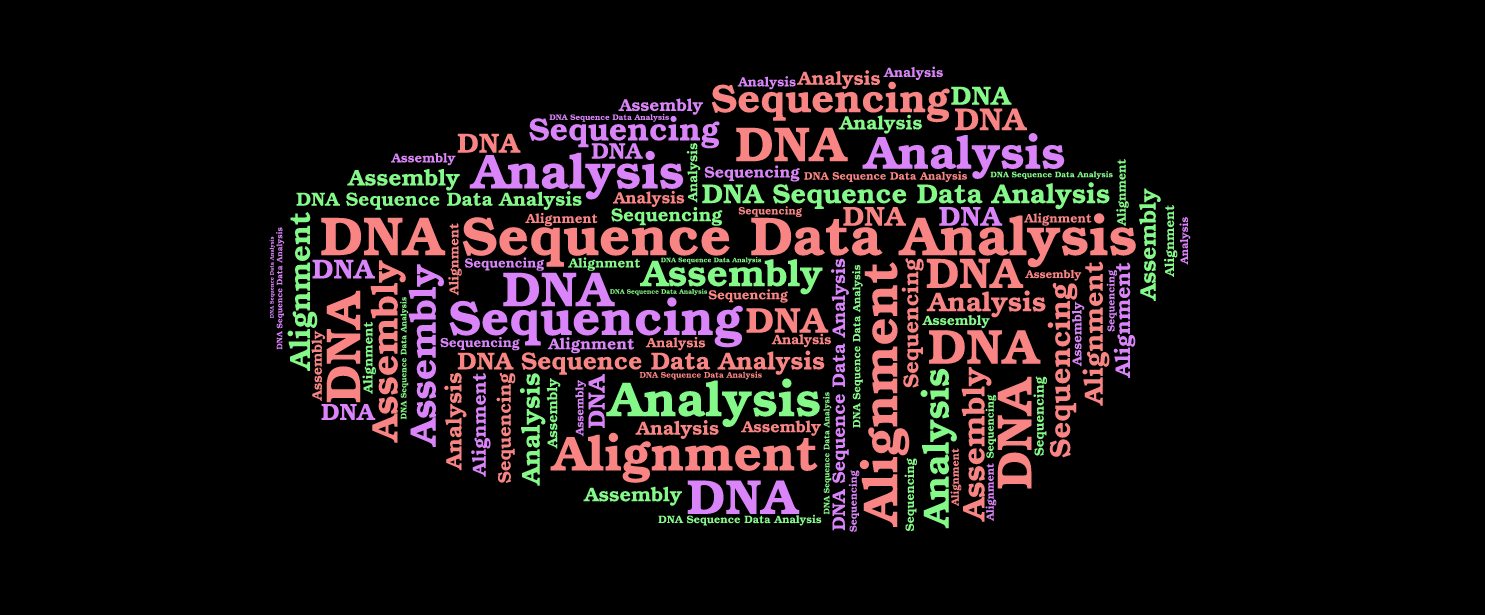Home Data Science High-Dimensional Data Analysis

# High-Dimensional Data Analysis

388
0A focus on several techniques that are widely used in the analysis of high-dimensional data.

Harvard University Online Course Highlights
• weeks long
• 2-4 hours per week
• Self-Paced
• Taught by: Rafael Irizarry, Michael Love
• View Course Syllabus## Online Course Details:

If you’re interested in data analysis and interpretation, then this is the data science course for you. We start by learning the mathematical definition of distance and use this to motivate the use of the singular value decomposition (SVD) for dimension reduction and multi-dimensional scaling and its connection to principle component analysis. We will learn about the batch effect: the most challenging data analytical problem in genomics today and describe how the techniques can be used to detect and adjust for batch effects. Specifically, we will describe the principal component analysis and factor analysis and demonstrate how these concepts are applied to data visualization and data analysis of high-throughput experimental data.

Finally, we give a brief introduction to machine learning and apply it to high-throughput data. We describe the general idea behind clustering analysis and descript K-means and hierarchical clustering and demonstrate how these are used in genomics and describe prediction algorithms such as k-nearest neighbors along with the concepts of training sets, test sets, error rates and cross-validation.

Given the diversity in educational background of our students we have divided the series into seven parts. You can take the entire series or individual courses that interest you. If you are a statistician you should consider skipping the first two or three courses, similarly, if you are biologists you should consider skipping some of the introductory biology lectures. Note that the statistics and programming aspects of the class ramp up in difficulty relatively quickly across the first three courses. By the third course will be teaching advanced statistical concepts such as hierarchical models and by the fourth advanced software engineering skills, such as parallel computing and reproducible research concepts.

These courses make up 2 XSeries and are self-paced:

• PH525.1x: Statistics and R for the Life Sciences
• PH525.2x: Introduction to Linear Models and Matrix Algebra
• PH525.3x: Statistical Inference and Modeling for High-throughput Experiments
• PH525.4x: High-Dimensional Data Analysis
• PH525.5x: Introduction to Bioconductor: annotation and analysis of genomes and genomic assays
• PH525.6x: High-performance computing for reproducible genomics
• PH525.7x: Case studies in functional genomics

This class was supported in part by NIH grant R25GM114818.

### What you’ll learn

• Mathematical Distance
• Dimension Reduction
• Singular Value Decomposition and Principal Component Analysis
• Multiple Dimensional Scaling Plots
• Factor Analysis
• Dealing with Batch Effects
• Clustering
• Heatmaps
• Basic Machine Learning Concepts